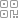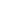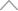5 9 7 0 4 4 3

# Python 中的单元测试框架和测试驱动开发 (TDD)

1. 介绍

2. 单元测试的重要性

3. 单元测试框架的一些基本功能

4. square 和 double 函数的单元测试

5. 命令行界面

6. 跳过单元测试和预期失败

7. 使用子集测试迭代import unittest

class StringMethodstest(unittest.TestCase):

def test_upper(self):

self.assertEqual('python'.upper(), 'PYTHON')

def test_isupper(self):

self.assertTrue('PYTHON'.isupper())

self.assertFalse('python'.isupper())

def test_split(self):

s = 'hello python'

self.assertEqual(s.split(), ['hello''python'])

with self.assertRaises(TypeError):

s.split(2)

if __name__ == '__main__':

unittest.main()

python -m unittest -v testpython函数中的单元测试示例：

def factorial(number):

if number == 0:

return 1

else:

return number * factorial(number-1)

import unittest

from mymodule1 import factorial

class TestFactorial(unittest.TestCase):

def test1(self):

self.assertEqual(factorial(4), 24# test when 4 is given as input the output is 24.

self.assertEqual(factorial(5), 120# test when 5 is given as input the output is 120.

self.assertEqual(factorial(0), 1)

unittest.main()

• 当输入 4 时，阶乘必须是 24。

• 当输入 5 时，阶乘必须是 120。

• 当输入 0 时，阶乘必须为 1。

• 当输入 2 时，输出不得为 2。

1. 最后一行中的 OK 表示所有测试都成功通过。

2. 最后一行的 FAILED 表明至少有一个测试失败，python 打印出哪些测试或测试失败。

![](http://qiniu.aihubs.net/12252unit 1 - 2.png)

import unittest

from mymodule1 import factorial

class TestFactorial(unittest.TestCase):

def test1(self):

self.assertEqual(factorial(4), 24)

self.assertEqual(factorial(5), 120)

self.assertNotEqual(factorial(2), 2)

unittest.main()

assertEqual() 的评估看起来像这样，失败并反馈失败的断言。

![](http://qiniu.aihubs.net/21752unit 1.png)考虑我们正在编写一个对数字进行平方和加倍的函数：

def square(number):

"""

This function returns the square of a number.

"""

return number ** 2

def double(number):

"""

This function returns twice the value of a number.

"""

return number * 2

square 和 double 函数的单元测试

1. 当输入 5 时，总和必须是 25。

2. 当输入 3.0 时，总和必须为 9.0。

3. 当输入 -3 时，总和不得为 -9。

4. 当输入 2 时，double 必须是 4。

5. 当输入-3.1 时，double 必须是-6.2。

6. 当输入 0 时，double 必须为 0。

import unittest

from mymodule import square, double, factorial

class TestSquare(unittest.TestCase):

def test1(self):

self.assertEqual(square(5), 25# test when 5 is given as input the output is 25.

self.assertEqual(square(3.0), 9.0)  # test when 3.0 is given as input the output is 9.0.

self.assertNotEqual(square(-3), -9)  # test when -3 is given as input the output is not -9.

class TestDouble(unittest.TestCase):

def test1(self):

self.assertEqual(double(2), 4# test when 2 is given as input the output is 4.

self.assertEqual(double(-3.1), -6.2# test when -3.1 is given as input the output is -6.2.

self.assertEqual(double(0), 0# test when 0 is given as input the output is 0.

unittest.main()

![](http://qiniu.aihubs.net/74662unit 2.png)

unittest 模块可以通过命令行界面从模块类甚至单个测试方法运行测试。我们可以传入一个包含任何模块名称序列以及完全限定的类或方法名称的列表。

python -m unittest test_mymodule1 test_module2

python -m unittest test_module.TestClass

python -m unittest test_module.TestClass.test_method

python -m unittest tests/test_mymodule.py

python -m unittest -v test_mymodule

python -m unittest

python -m unittest -h

class MyTestCase(unittest.TestCase):

@unittest.skipUnless(sys.platform.startswith("win"), "requires Windows")

def test_windows_support(self):

# windows specific testing code

pass

def test_maybe_skipped(self):

if not external_resource_available():

self.skipTest("external resource not available")

# test code that depends on the external resource

pass

@unittest.skip("showing class skipping")

class SkippedTestCase(unittest.TestCase):

def test_not_run(self):

pass

class ExpectedFailureTestCase(unittest.TestCase):

@unittest.expectedFailure

def test_fail(self):

self.assertEqual(10"broken")

class TestNumbers(unittest.TestCase):

def test_even(self):

"""

Test that numbers between 0 and 6 are all even.

"""

for i in range(07):

with self.subTest(i=i):

self.assertEqual(i % 20)

## 新知精选

IT研发知识▪优秀创作者

### 优选课程 新知学院

•扫码下载商业新知APP~

•回到顶部